# Cell type classification with SignacX: CITE-seq PBMCs from 10X Genomics

#### 2021-02-26

This vignette shows how to use Signac with Seurat. There are three parts: Seurat, Signac and then visualization. We use an example PBMCs CITE-seq data set from 10X Genomics.

## Seurat

library(Seurat)

dir.create("fls")
destfile = "fls/pbmc_10k_protein_v3_filtered_feature_bc_matrix.h5")

Create a Seurat object, and then perform SCTransform normalization. Note:

• You can use the legacy functions here (i.e., NormalizeData, ScaleData, etc.), use SCTransform or any other normalization method (including no normalization). We did not notice a significant difference in cell type annotations with different normalization methods.
• We think that it is best practice to use SCTransform, but it is not a necessary step. SignacX will work fine without it.
# load dataset
pbmc <- CreateSeuratObject(counts = E$Gene Expression, project = "pbmc") # run sctransform pbmc <- SCTransform(pbmc) Perform dimensionality reduction by PCA and UMAP embedding. Note: • SignacX actually needs these functions since it uses the nearest neighbor graph generated by Seurat. # These are now standard steps in the Seurat workflow for visualization and clustering pbmc <- RunPCA(pbmc, verbose = FALSE) pbmc <- RunUMAP(pbmc, dims = 1:30, verbose = FALSE) pbmc <- FindNeighbors(pbmc, dims = 1:30, verbose = FALSE) ## SignacX Load the package require(SignacX) Generate SignacX labels for the Seurat object. Note: • Optionally, you can do parallel computing by setting num.cores > 1 in the Signac function. • Run time is ~10-20 minutes for <100,000 cells. # Run Signac labels <- Signac(pbmc, num.cores = 4) celltypes = GenerateLabels(labels, E = pbmc) Can we make Signac faster? Sometimes, training the neural networks takes a lot of time. To make Signac faster, we implemented SignacFast which uses an ensemble of pre-trained neural network models. Note: • SignacFast uses an ensemble of 1,800 pre-calculated neural networks using the GenerateModels function together with the training_HPCA reference data. • Features that are absent from the single cell data and present in the neural network are set to zero. # Run Signac labels_fast <- SignacFast(pbmc) celltypes_fast = GenerateLabels(labels_fast, E = pbmc) SignacFast took only ~30 seconds. Relative to Signac, the main difference is that SignacFast tends to leave a few more cells “Unclassified.” How does SignacFast compare to Signac? B MPh TNK Unclassified B 550 0 0 0 MPh 0 2178 0 0 TNK 0 0 4914 0 Unclassified 0 4 2 217 ## Visualizations Now we can visualize the cell type classifications at many different levels: Immune and nonimmune pbmc <- AddMetaData(pbmc, metadata = celltypes_fast$Immune, col.name = "immmune")
pbmc <- SetIdent(pbmc, value = "immmune")
png(filename = "fls/plot1_citeseq.png")
DimPlot(pbmc)
dev.off()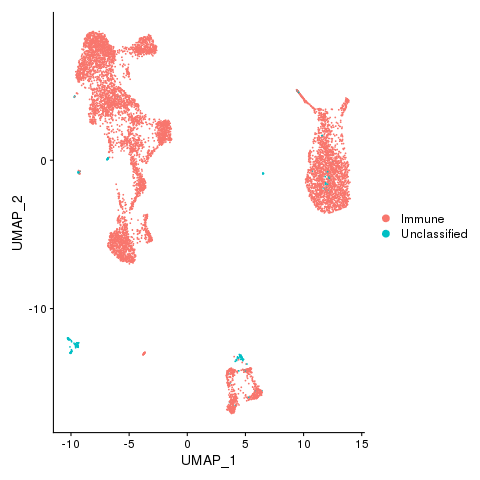Immune, Nonimmune (if any) and unclassified cells

pbmc <- AddMetaData(pbmc, metadata = celltypes$L2, col.name = "celltypes") pbmc <- SetIdent(pbmc, value = "celltypes") png(filename = "fls/plot2_citeseq.png") DimPlot(pbmc) dev.off()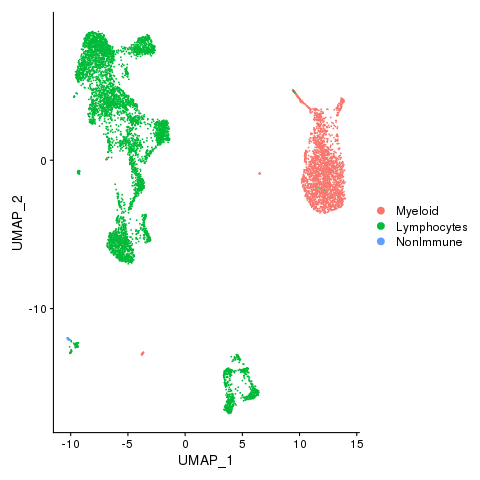Myeloid and lymphocytes pbmc <- AddMetaData(pbmc, metadata = celltypes$CellTypes, col.name = "celltypes")
pbmc <- SetIdent(pbmc, value = "celltypes")
png(filename = "./fls/plot3_citeseq.png")
DimPlot(pbmc)
dev.off()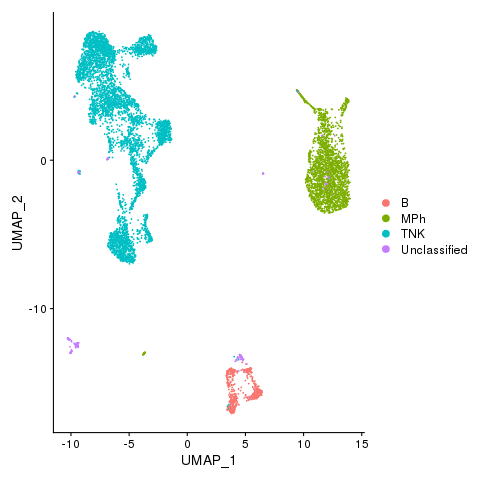Cell types

pbmc <- AddMetaData(pbmc, metadata = celltypes$CellTypes_novel, col.name = "celltypes_novel") pbmc <- SetIdent(pbmc, value = "celltypes_novel") png(filename = "./fls/plot4_citeseq.png") DimPlot(pbmc) dev.off()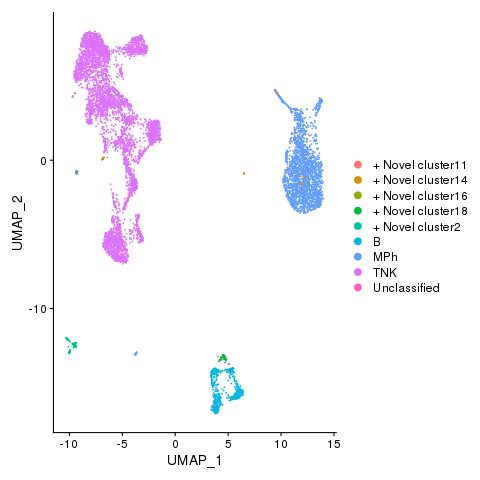Cell types with novel populations pbmc <- AddMetaData(pbmc, metadata = celltypes$CellStates, col.name = "cellstates")
pbmc <- SetIdent(pbmc, value = "cellstates")
png(filename = "./fls/plot5_citeseq.png")
DimPlot(pbmc)
dev.off()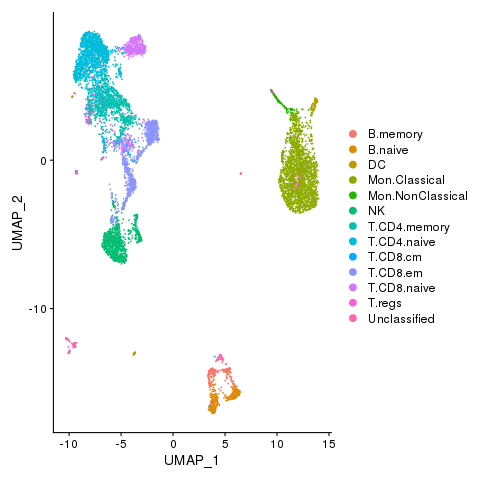Cell states

Identify differentially expressed genes between cell types.

pbmc <- SetIdent(pbmc, value = "celltypes")

# Find markers for all clusters, and draw a heatmap
markers <- FindAllMarkers(pbmc, only.pos = TRUE, verbose = F, logfc.threshold = 1)
library(dplyr)
top5 <- markers %>% group_by(cluster) %>% top_n(n = 5, wt = avg_logFC)

png(filename = "./fls/plot9_citeseq.png", width = 640, height = 720)
DoHeatmap(pbmc, features = unique(top5$gene), angle = 90) dev.off()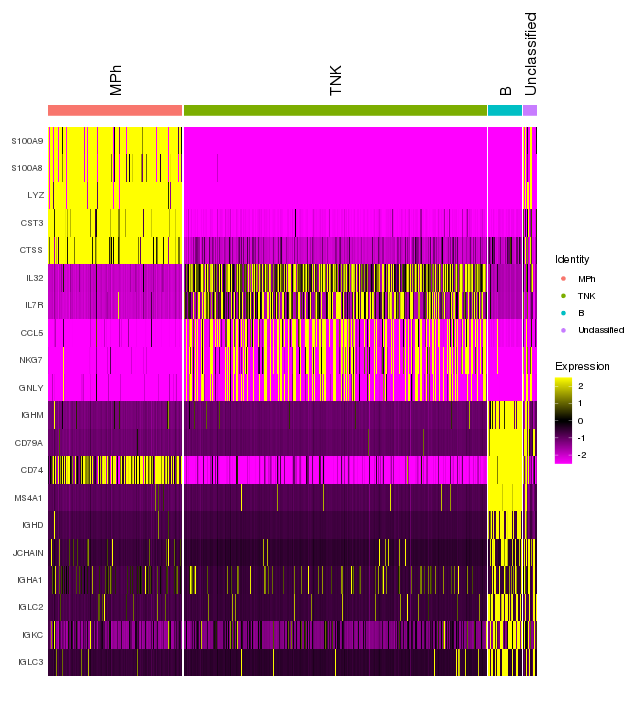Immune marker genes - cell types pbmc <- SetIdent(pbmc, value = "cellstates") # Find markers for all clusters, and draw a heatmap markers <- FindAllMarkers(pbmc, only.pos = TRUE, verbose = F, logfc.threshold = 1) top5 <- markers %>% group_by(cluster) %>% top_n(n = 5, wt = avg_logFC) png(filename = "./fls/plot6_citeseq.png", width = 640, height = 720) DoHeatmap(pbmc, features = unique(top5$gene), angle = 90)
dev.off()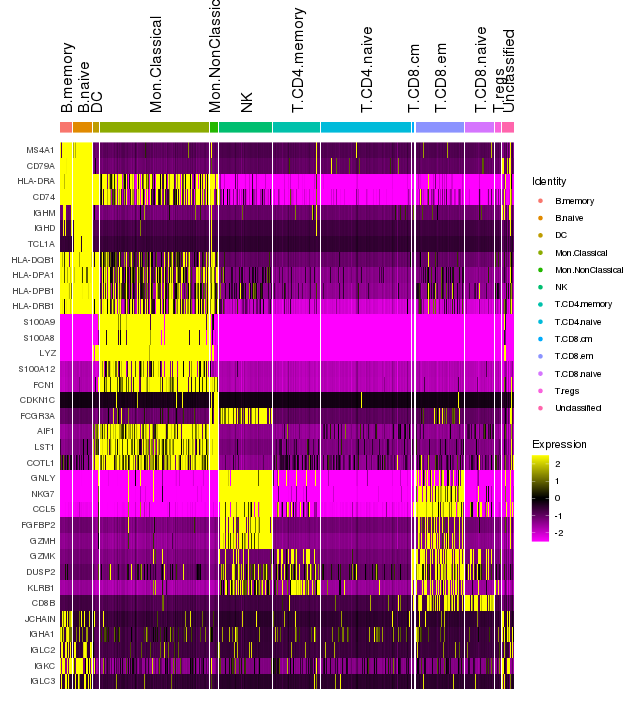Add protein expression information

pbmc[["ADT"]] <- CreateAssayObject(counts = E$Antibody Capture[, colnames(E$Antibody Capture) %in%
colnames(pbmc)])
pbmc <- NormalizeData(pbmc, assay = "ADT", normalization.method = "CLR")
pbmc <- ScaleData(pbmc, assay = "ADT")

Identify differentially expressed proteins between clusters

DefaultAssay(pbmc) <- "ADT"
# Find protein markers for all clusters, and draw a heatmap
adt.markers <- FindAllMarkers(pbmc, assay = "ADT", only.pos = TRUE, verbose = F)
png(filename = "./fls/plot7_citeseq.png", width = 640, height = 720)
DoHeatmap(pbmc, features = unique(adt.markers\$gene), angle = 90)
dev.off()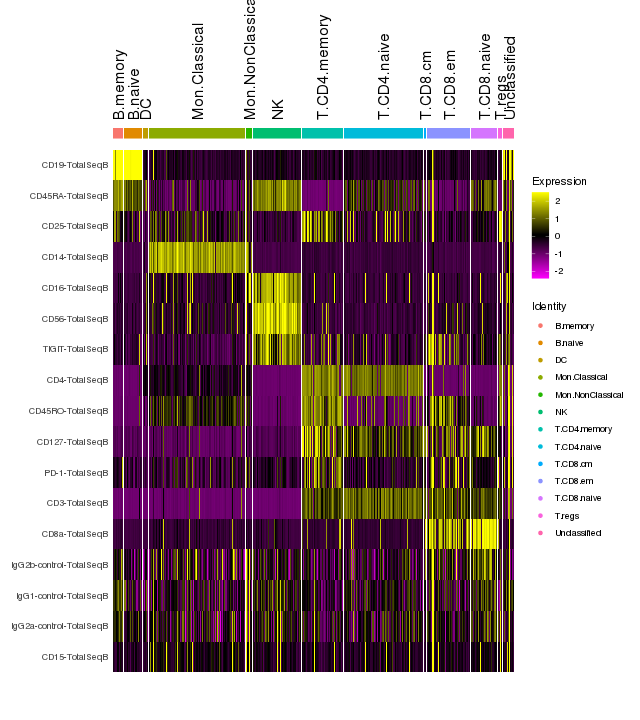Immune marker genes

Save results

saveRDS(pbmc, file = "fls/pbmcs_signac_citeseq.rds")
saveRDS(celltypes, file = "fls/celltypes_citeseq.rds")
saveRDS(celltypes_fast, file = "fls/celltypes_fast_citeseq.rds")

Session Info

## R version 3.5.0 (2018-04-23)
## Platform: x86_64-pc-linux-gnu (64-bit)
## Running under: CentOS Linux 7 (Core)
##
## Matrix products: default
## BLAS/LAPACK: /site/ne/home/i0369218/.local/share/r-miniconda/envs/r-reticulate/lib/libopenblasp-r0.3.10.so
##
## locale:
##   LC_CTYPE=en_US.UTF-8       LC_NUMERIC=C
##   LC_TIME=en_US.UTF-8        LC_COLLATE=C
##   LC_MONETARY=en_US.UTF-8    LC_MESSAGES=en_US.UTF-8
##   LC_PAPER=en_US.UTF-8       LC_NAME=C
##  digest_0.6.18   xfun_0.12       rlang_0.4.8     evaluate_0.14